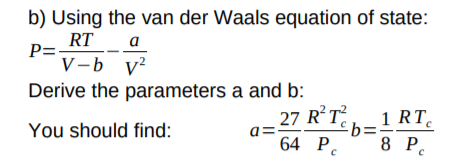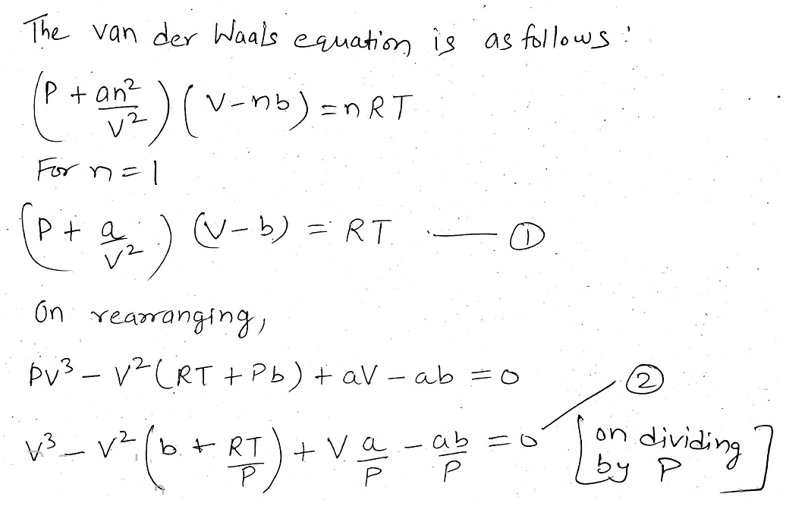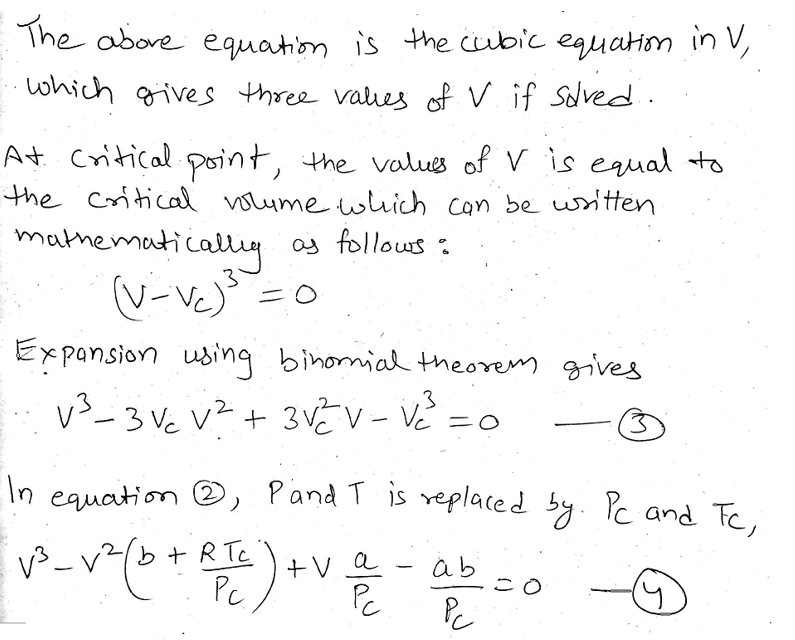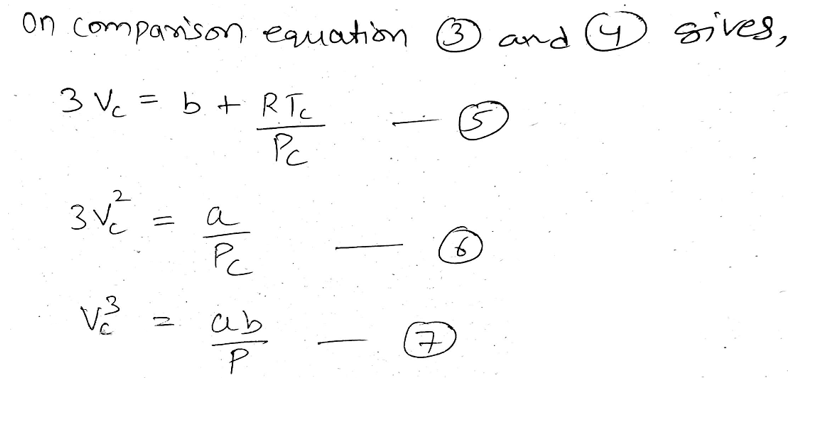# b) Using the van der Waals equation of state:P= RTV-b v?aDerive the parameters a and b:27 RT£b=:64 P.1 RTYou should find:8 P.

Question
1 views

Using the Van de Waals equation of state, derive the parameters a and b.help_outlineImage Transcriptioncloseb) Using the van der Waals equation of state: P= RT V-b v? a Derive the parameters a and b: 27 RT £b=: 64 P. 1 RT You should find: 8 P. fullscreen
check_circle

Step 1

The rearrangement of the van der Waals equation gives the following equations.Step 2

The solution of the above cubic equation in V provides three values of V.Step 3

The comparison of equation (3) and (4) provides the following relations....

### Want to see the full answer?

See Solution

#### Want to see this answer and more?

Solutions are written by subject experts who are available 24/7. Questions are typically answered within 1 hour.*

See Solution
*Response times may vary by subject and question.
Tagged in